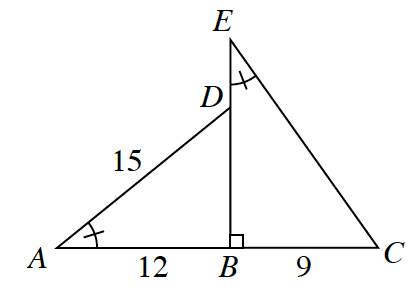### Home > CCG > Chapter 4 > Lesson 4.2.5 > Problem4-118

4-118.Examine the diagram at right. If $\overline{AC}$ passes through point $B$, then answer the questions below.

1. Are the triangles similar? If so, make a flowchart justifying your answer.

What do the tick marks on the angles mean? What is $m∠B$? How can these help you determine if the triangles are similar?

2. Are the triangles congruent? Explain how you know.

Use the Pythagorean Theorem to calculate the length of $\overline{BD}$.